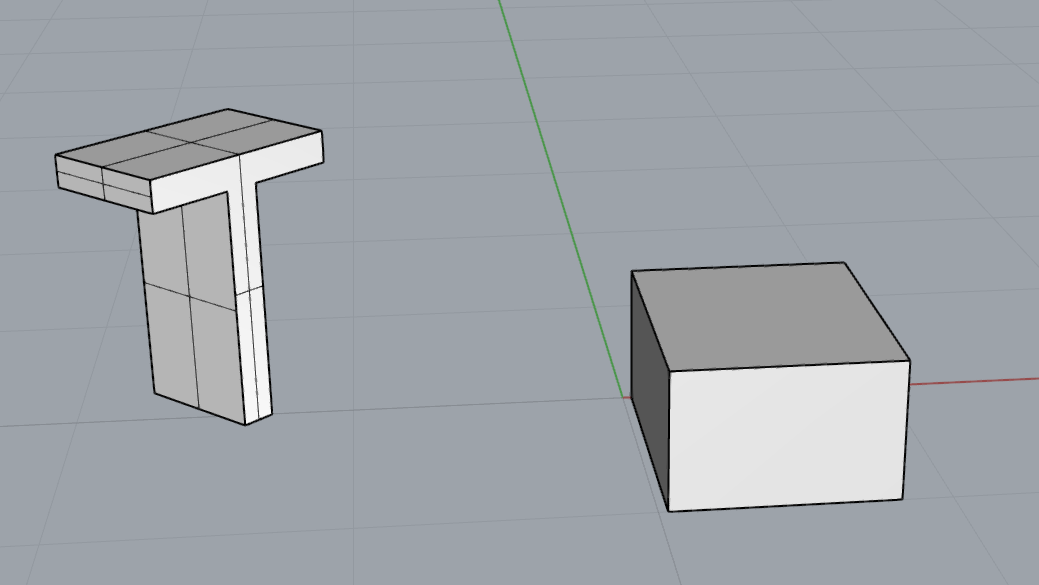# Unexpected result from Brep.GetBoundingBox(Plane plane) of text geometry

Hello everyone,

I have used Brep.GetBoundingBox(Plane plane) before. However, when I use it on a brep created from a text entity, it fails me:

``````from Rhino import *
from Rhino.DocObjects import *
from Rhino.Geometry import *

doc = RhinoDoc.ActiveDoc
doc.Objects.Clear()

text = "T"
style = DimensionStyle()
plane = Plane(Point3d(-2.0, 0.0, 1.0), Point3d(-1.0, 1.0, 1.0), Point3d(-2.0, 0.0, 2.0))

entity = TextEntity.CreateWithRichText(text, plane, style, False, 10.0, 0.0)

brep = entity.CreatePolySurfaces(style, 0.5)
bbox = Box(brep.GetBoundingBox(plane))

doc.Views.Redraw()
``````

In the code above I create some text aligned to a plane in space. When I try to get the plane-aligned bounding box of this text, things go wrong. What I get in fact, is the bounding box of the text as if it were created in Plane.WorldXY. Here is an example picture:I do not understand how I get this result. Am I missing something, or is it a bug?

Greeting from Berlin,
Thomas

Hi @thomas.gust,

The problem is this line:

`````` bbox = Box(brep.GetBoundingBox(plane))
``````

The box constructor has an overload that takes a plane, I you don’t supply any `Plane.WorldXY` is used. See https://developer.rhino3d.com/api/RhinoCommon/html/M_Rhino_Geometry_Box__ctor_1.htm

Your line should look something like this:

`````` bbox = Box(plane, brep.GetBoundingBox(plane))
``````

Thank you @lando.schumpich, that does the trickMy error was to think of the bounding box in global coordinates, while it was in coordinates relative to the plane.

There is even another overload for the bounding box that computes directly a box in global coordinates:
https://developer.rhino3d.com/api/RhinoCommon/html/M_Rhino_Geometry_GeometryBase_GetBoundingBox_1.htm